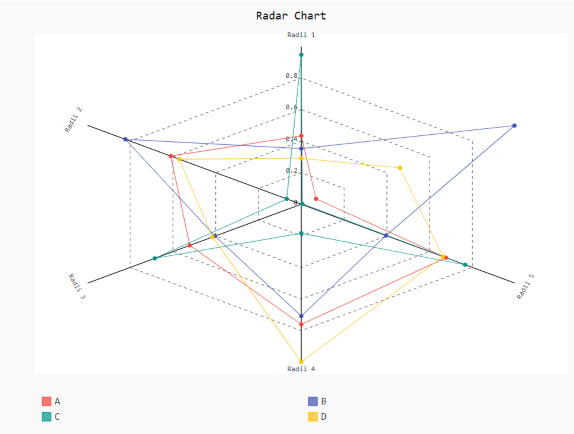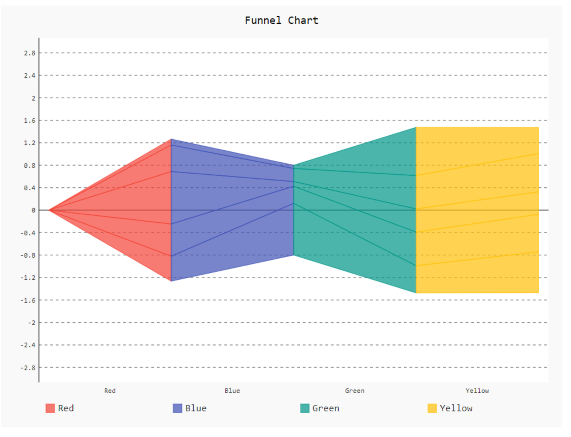GeeksforGeeks App
Open AppBrowser
Continue

# How to change the position of legend in Pygal?

Prerequisites:  pygal

Pygal is a Python module that is mainly used to build SVG (Scalar Vector Graphics) graphs and charts. Pygal is a graphics and user interface library for Python that provides functionality commonly required in designing and science applications.
In this article, we will see how we can change the legend position and number of legend columns in the plot window. While making a plot it is important for us to optimize its size and legend position. Here are various ways to change the default legend position as per our requirement.

Approach:

• Import required module.
• Create a chart object.
• Pass legend position in the same function.
• Label the graph.
• Display Graph.

Implementation of the concept discussed above is given below:

Example 1:

## Python3

 `# importing pygal``import` `pygal``import` `numpy`` ` `# creating the chart object``Radar_Chart ``=` `pygal.Radar(legend_at_bottom``=``True``)`` ` ` ` `Radar_Chart.x_labels ``=` `[``'Radii 1'``, ``'Radii 2'``,``                        ``'Radii 3'``, ``'Radii 4'``,``                        ``'Radii 5'``]`` ` `# Random data``Radar_Chart.add(``'A'``, numpy.random.rand(``6``))``Radar_Chart.add(``'B'``, numpy.random.rand(``6``))``Radar_Chart.add(``'C'``, numpy.random.rand(``6``))``Radar_Chart.add(``'D'``, numpy.random.rand(``6``))`` ` `# naming the title``Radar_Chart.title ``=` `'Radar Chart'`` ` `Radar_Chart.render_to_png(``'aa.png'``)`

OutputExample 2:

## Python3

 `# importing pygal``import` `pygal``import` `numpy`` ` ` ` `# creating the chart object``funnel ``=` `pygal.Funnel(legend_at_bottom``=``True``, legend_at_bottom_columns``=``4``)`` ` `# Random data``funnel.add(``'Red'``, numpy.random.rand(``5``))``funnel.add(``'Blue'``, numpy.random.rand(``5``))``funnel.add(``'Green'``, numpy.random.rand(``5``))``funnel.add(``'Yellow'``, numpy.random.rand(``5``))`` ` ` ` `# naming the title``funnel.title ``=` `'Funnel Chart'`` ` `funnel.render_to_png(``'aa.png'``)`

OutputMy Personal Notes arrow_drop_up